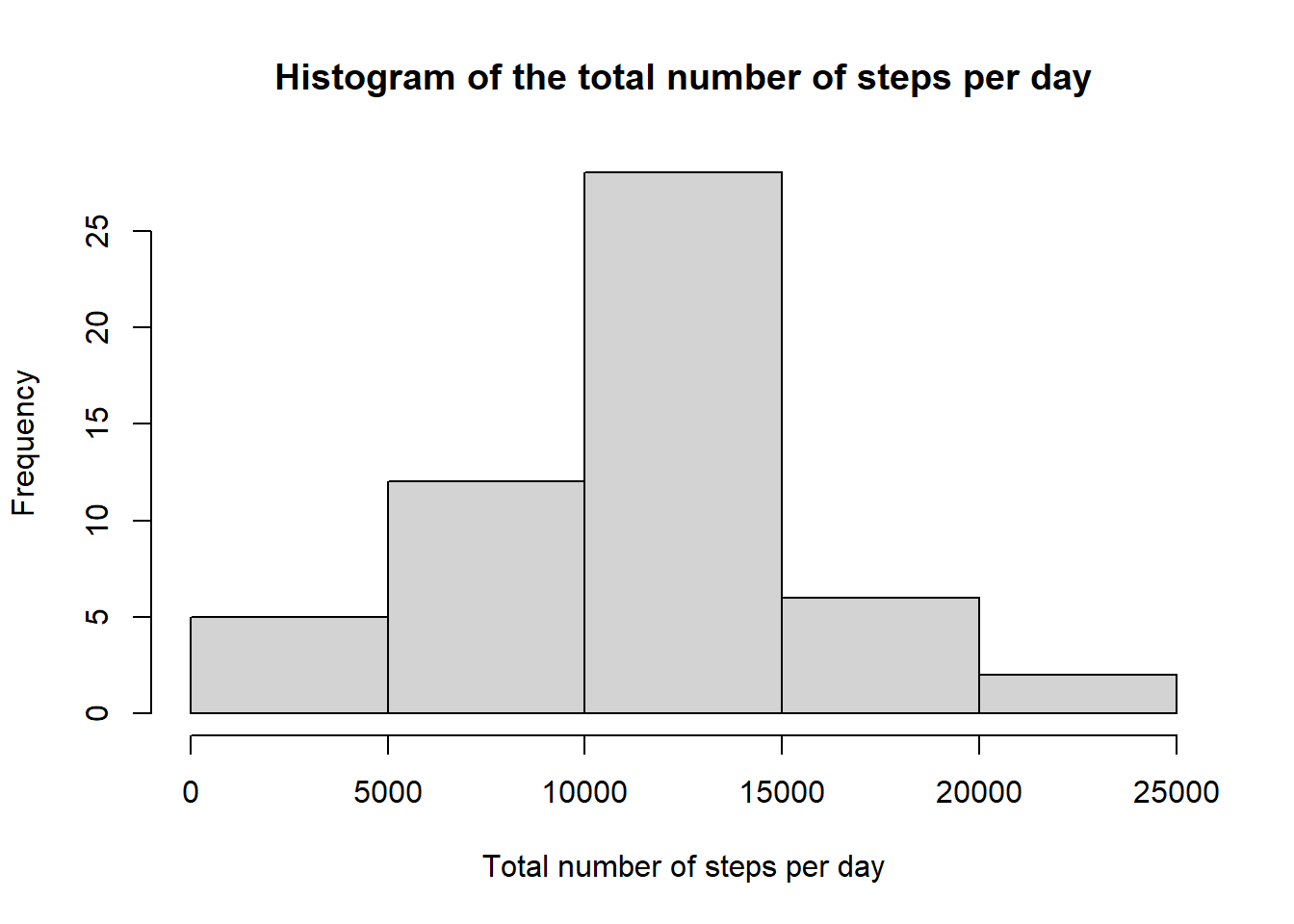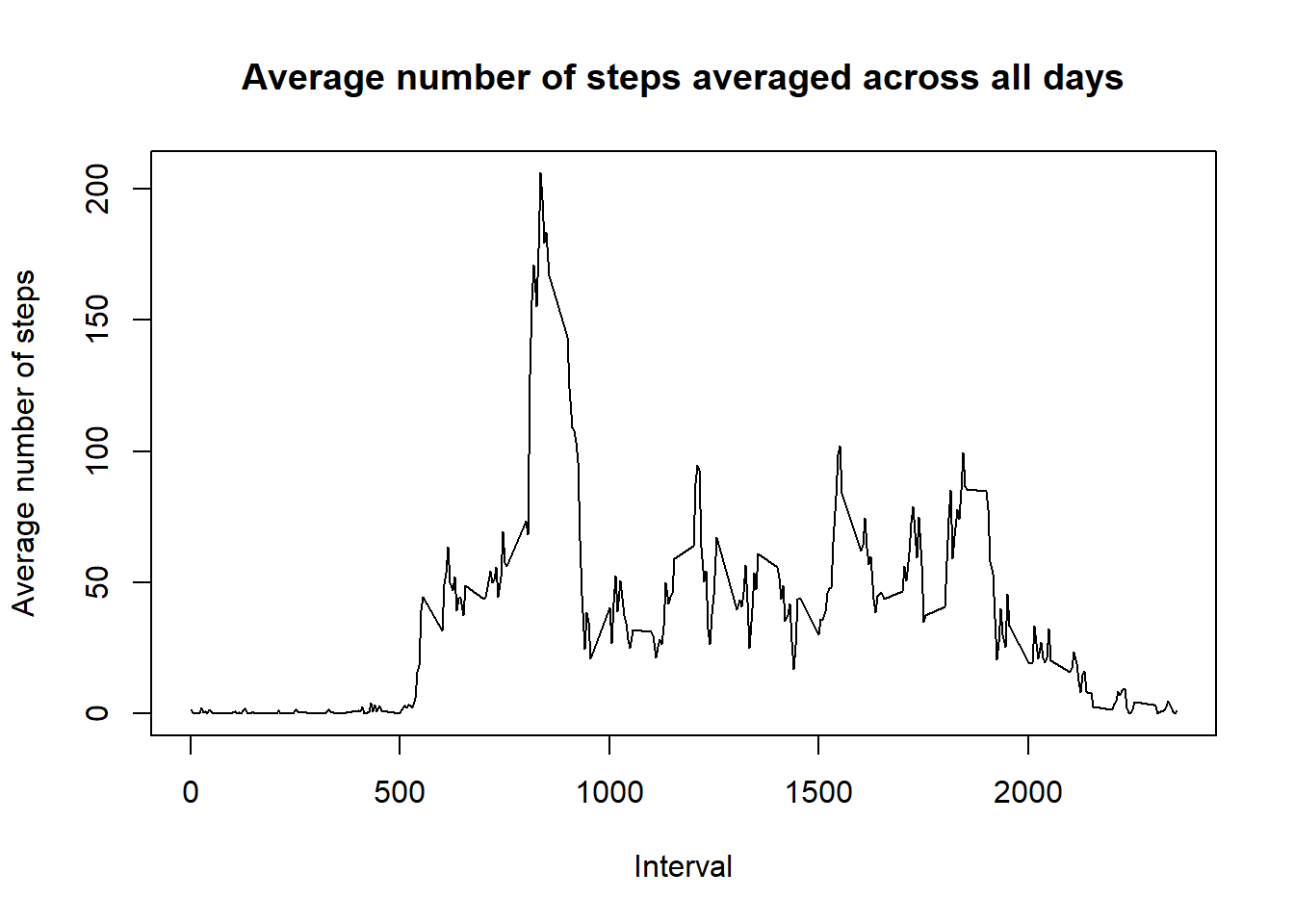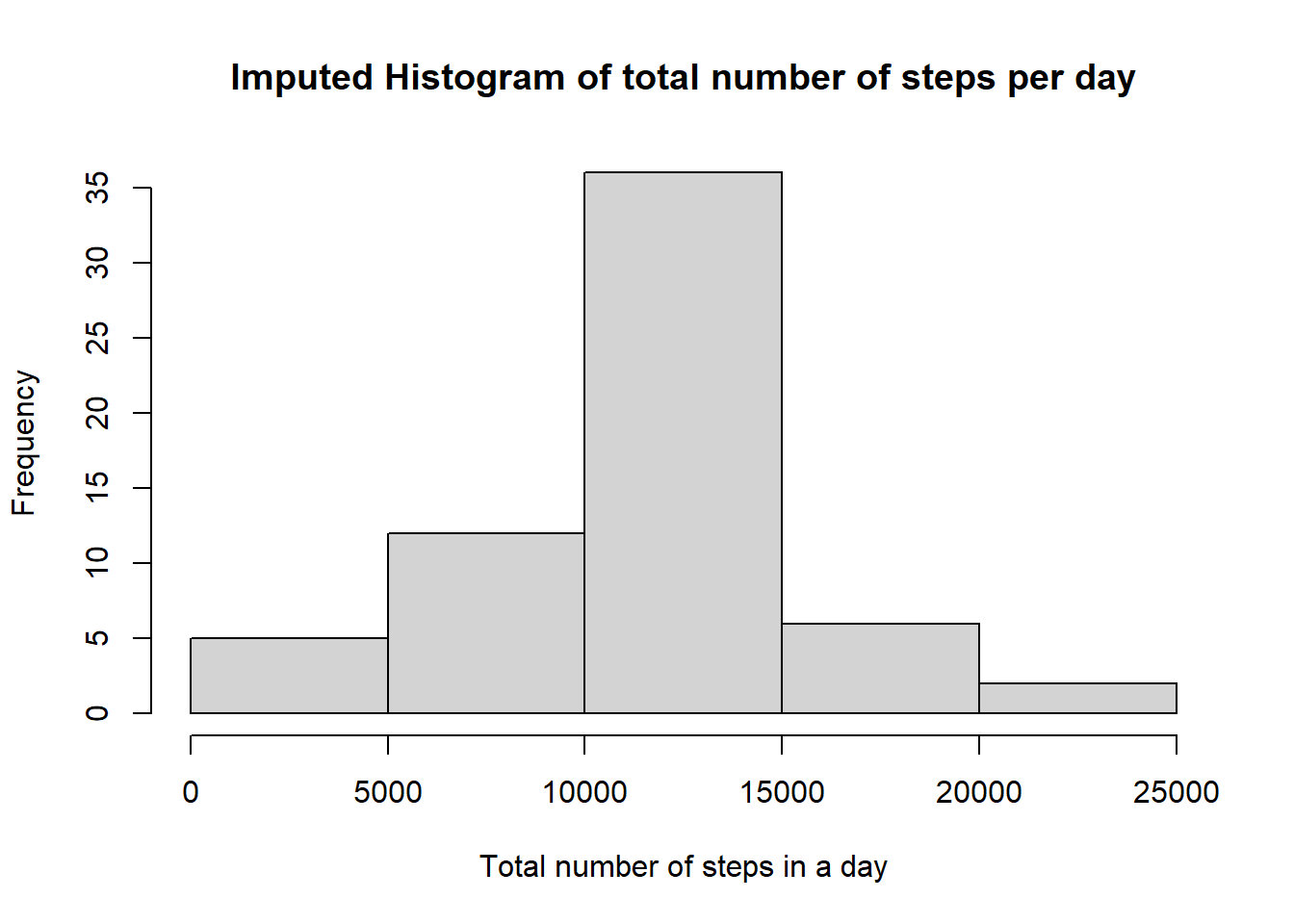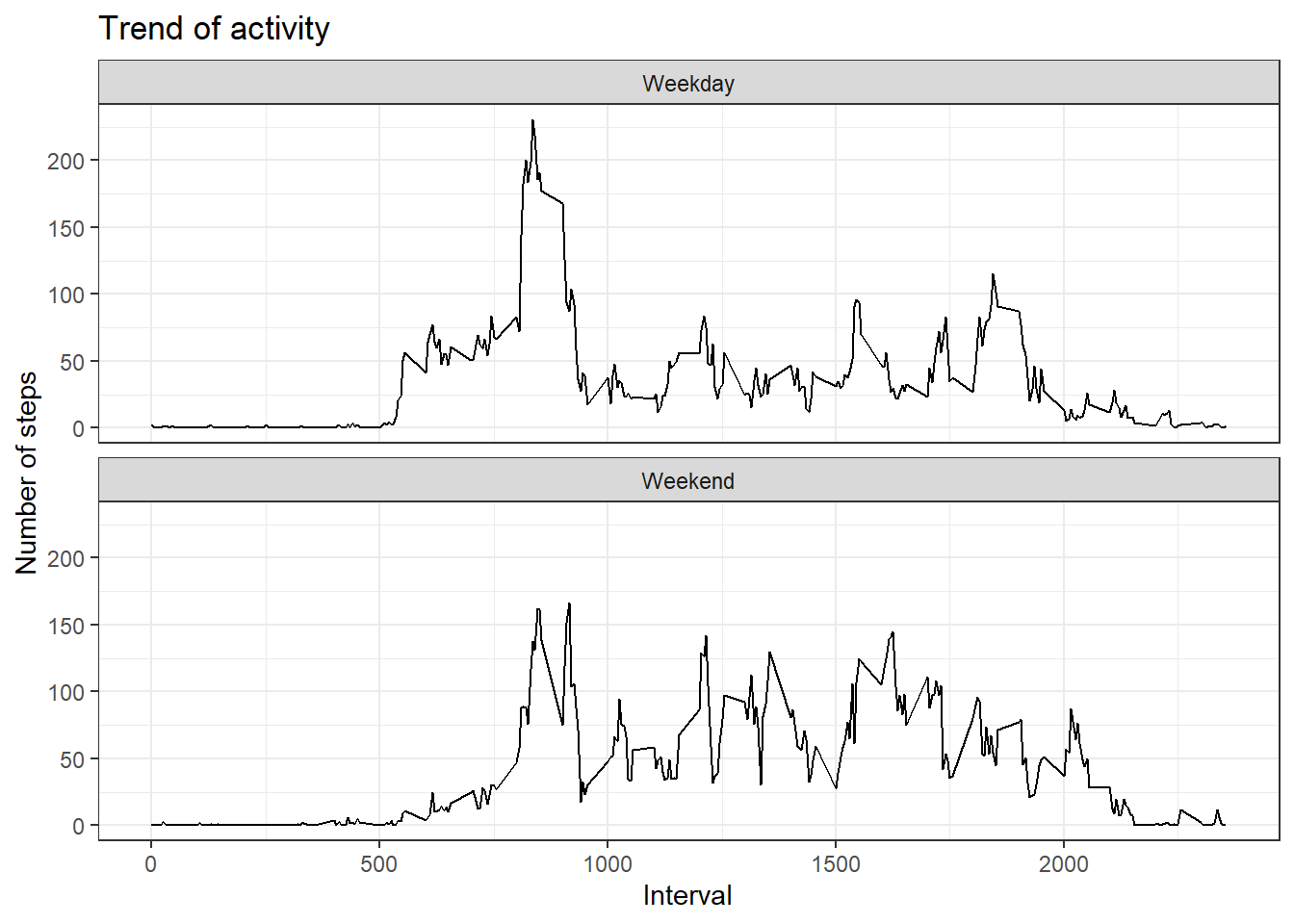## R Markdown

This document is created as part of Peer-graded Assignment: Course Project 1 of Coursera course Reproducible Research offered by Johns Hopkins University and facilitated by Dr. Roger D. Peng https://www.coursera.org/learn/reproducible-research.

The analysis was carried out in R and was based on “quantified self” movement data from a personal activity monitoring device. This device collects data at 5 minute intervals through out the day. The data consists of two months of data from an anonymous individual collected during the months of October and November, 2012 and include the number of steps taken in 5 minute intervals each day. This document was created using Knit and R Markdown.

## Load, explore and preprocess the data

#load
#explore
names(act)
##  "steps"    "date"     "interval"
str(act)
## 'data.frame':    17568 obs. of  3 variables:
##  $steps : int NA NA NA NA NA NA NA NA NA NA ... ##$ date    : chr  "2012-10-01" "2012-10-01" "2012-10-01" "2012-10-01" ...
##  $interval: int 0 5 10 15 20 25 30 35 40 45 ... summary(act) ## steps date interval ## Min. : 0.00 Length:17568 Min. : 0.0 ## 1st Qu.: 0.00 Class :character 1st Qu.: 588.8 ## Median : 0.00 Mode :character Median :1177.5 ## Mean : 37.38 Mean :1177.5 ## 3rd Qu.: 12.00 3rd Qu.:1766.2 ## Max. :806.00 Max. :2355.0 ## NA's :2304 #omit NAs act1 <- na.omit(act) str(act1) ## 'data.frame': 15264 obs. of 3 variables: ##$ steps   : int  0 0 0 0 0 0 0 0 0 0 ...
##  $date : chr "2012-10-02" "2012-10-02" "2012-10-02" "2012-10-02" ... ##$ interval: int  0 5 10 15 20 25 30 35 40 45 ...
##  - attr(*, "na.action")= 'omit' Named int [1:2304] 1 2 3 4 5 6 7 8 9 10 ...
##   ..- attr(*, "names")= chr [1:2304] "1" "2" "3" "4" ...

## Mean total number of steps taken per day

library(dplyr)
##
## Attaching package: 'dplyr'
## The following objects are masked from 'package:stats':
##
##     filter, lag
## The following objects are masked from 'package:base':
##
##     intersect, setdiff, setequal, union
day_step <- act1%>%
group_by(date)%>%
summarise(tot_steps=sum(steps))
## summarise() ungrouping output (override with .groups argument)
str(day_step)            
## tibble [53 x 2] (S3: tbl_df/tbl/data.frame)
##  $date : chr [1:53] "2012-10-02" "2012-10-03" "2012-10-04" "2012-10-05" ... ##$ tot_steps: int [1:53] 126 11352 12116 13294 15420 11015 12811 9900 10304 17382 ...
#1) a histogram of the total number of steps taken each day
hist(day_step$tot_steps, main="Histogram of the total number of steps per day", xlab="Total number of steps per day")#2.a) Mean of steps mean1<- mean(day_step$tot_steps)
#2.b) Mediann of steps
median1 <- median(day_step$tot_steps) The mean is 1.076618910^{4} and the median is 10765. ## The average daily activity pattern? Make a time series plot (i.e. type = “l”) of the 5-minute interval (x-axis) and the average number of steps taken, averaged across all days (y-axis). library(dplyr) inter_step <- aggregate(steps ~ interval, act, mean) plot(inter_step$interval, inter_step$steps, type='l', main="Average number of steps averaged across all days", xlab="Interval", ylab="Average number of steps")# find row id of maximum average number of steps in an interval max_row_id <- which.max(inter_step$steps)

# get the interval with maximum average number of steps in an interval
inter_step [max_row_id, ]
##     interval    steps
## 104      835 206.1698

## Imputing missing values

1. Calculate and report the total number of missing values in the dataset (i.e. the total number of rows with NAs).
act_NA <- act[!complete.cases(act),]

# number of rows
NArows <- nrow(act_NA)

The number of rows with NAs is 2304. ## Filling in the missing values

# Create a new dataset that is equal to the original dataset but with the missing data filled in.
for (i in 1:nrow(act)){
if (is.na(act$steps[i])){ interval_val <- act$interval[i]
row_id <- which(inter_step$interval == interval_val) steps_val <- inter_step$steps[row_id]
act$steps[i] <- steps_val } } Make a histogram of the total number of steps taken each day and Calculate and report the mean and median total number of steps taken per day. # aggregate steps as per date to get total number of steps in a day inserted <- aggregate(steps ~ date, act, sum) # create histogram of total number of steps in a day hist(inserted$steps, main="Imputed Histogram of total number of steps per day", xlab="Total number of steps in a day")mean2 <- mean(inserted$steps) median2 <- median(inserted$steps)

Mean with imputed values is 1.076618910^{4} whereas previously it was 1.076618910^{4}. The median with imputed values is 1.076618910^{4} whereas it was 10765 before.

## Differences in activity patterns between weekdays and weekends

Create a new factor variable in the dataset with two levels – “weekday” and “weekend”

# Create a new factor variable in the dataset with two levels – “weekday” and “weekend” indicating whether a given date is a weekday or weekend day.
day <- weekdays(as.Date(act$date)) daylevel <- vector() for (i in 1:nrow(act)) { if (day[i] == "Saturday") { daylevel[i] <- "Weekend" } else if (day[i] == "Sunday") { daylevel[i] <- "Weekend" } else { daylevel[i] <- "Weekday" } } act$daylevel <- daylevel
act$daylevel <- factor(act$daylevel)

stepsByDay <- aggregate(steps ~ interval + daylevel, data = act, mean)
names(stepsByDay) <- c("interval", "daylevel", "steps")

Make a panel plot containing a time series plot (i.e. type = “l”) of the 5-minute interval (x-axis) and the average number of steps taken, averaged across all weekday days or weekend days (y-axis).

# make the panel plot for weekdays and weekends
library(ggplot2)
# create the panel plot
ggplot(stepsByDay, aes(x=interval, y=steps)) +
geom_line(linetype=1) +
theme_bw() +
facet_wrap(vars(daylevel), nrow = 2)+
ggtitle("Trend of activity")+
xlab("Interval")+
ylab("Number of steps")